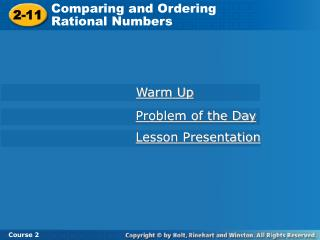Download Presentation2-11

# 2-11 - PowerPoint PPT PresentationDownload Presentation## 2-11

- - - - - - - - - - - - - - - - - - - - - - - - - - - E N D - - - - - - - - - - - - - - - - - - - - - - - - - - -
##### Presentation Transcript

1. Comparing and Ordering Rational Numbers 2-11 Course 2 Warm Up Problem of the Day Lesson Presentation

2. Comparing and Ordering Rational Numbers 2-11 Course 2 Warm Up Write each mixed number as an improper fraction. 1.1 3. 1 3 4 7 8 2. 2 1 12 5 6 4. 8

3. 2-10 Equivalent Fractions and Decimals Course 2 In baseball, a player’s batting average compares the number of hits with the number of times the player has been at bat. The statistics on the next slide are for the 2004 Major League Baseball season.

4. 2-10 Equivalent Fractions and Decimals Course 2 Hits at Bats 172 544 544 172 ÷ 544 ≈ 0.316 172 172 601 172 601 172 ÷ 601 ≈ 0.286 To convert a fraction to a decimal, divide the numerator by the denominator.

5. 2-10 Equivalent Fractions and Decimals Course 2 Write each fraction as a decimal. Round to the nearest hundredth, if necessary. Example: 9 5 1 4 B. A. These are called Terminating Decimals Because the decimals come to an end.

6. 2-10 Equivalent Fractions and Decimals Course 2 Insert Lesson Title Here Write each fraction as a decimal. Round to the nearest hundredth, if necessary. Examples: 5 3 7 3 e. f. Repeating Decimals These are called Because the decimal repeats the pattern forever.

7. 2-10 Equivalent Fractions and Decimals Course 2 You should write a repeating decimal with a bar over the repeating part. For example: 0.333… = 0.3 0.8333… = 0.83 0.727272… = 0.72

8. 2-10 Equivalent Fractions and Decimals Course 2 Use Mental Math to Write Fractions as Decimals Write each fraction as a decimal. 4 5 3 5 A. c. 37 50 18 25 B. d.

9. 2-10 Equivalent Fractions and Decimals Reading Math You read the decimal 0.018 as “eighteen thousandths.” Course 2 Write each decimal as a fraction in simplest form. B. 1.55 A. 0.018

10. 2-10 Equivalent Fractions and Decimals Course 2 Insert Lesson Title Here Write each decimal as a fraction in simplest form. D. 1.30 C. 0.015

11. Comparing and Ordering Rational Numbers 2-11 Course 2 Learn to compare and order fractions and decimals.

12. Comparing and Ordering Rational Numbers 2-11 Course 2 Insert Lesson Title Here Vocabulary rational number

13. Comparing and Ordering Rational Numbers 2-11 Course 2 7 9 2 9 Which is greater, or ? When two fractions have the same denominator, just compare the numerators. 7 9 2 9 > because 7 > 2. 7 9 = 2 9 =

14. Comparing and Ordering Rational Numbers 2-11 7 9 11 15 Course 2 Compare the fractions. Write < or >. Using LCM

15. Comparing and Ordering Rational Numbers 2-11 2 5 3 7 Course 2 Compare the fractions. Write < or >. – – Using LCM

16. Comparing and Ordering Rational Numbers 2-11 5 6 7 8 Course 2 Now, YOU try! Compare the fractions. Write < or >.

17. Comparing and Ordering Rational Numbers 2-11 6 8 5 7 Course 2 Now, YOU try! Compare the fractions. Write < or >. – –

18. Comparing and Ordering Rational Numbers 2-11 Course 2 To compare decimals, line up the decimal points and compare digits from left to right until you find the place where the digits are different.

19. Comparing and Ordering Rational Numbers 2-11 Course 2 Compare the decimals. Write < or >. 0.427 0.425 Line up and check from left to right

20. Comparing and Ordering Rational Numbers 2-11 Course 2 Compare the decimals. Write < or >. 0.73 0.734

21. Comparing and Ordering Rational Numbers 2-11 Course 2 Now, YOU try! Compare the decimals. Write < or >. 0.535 0.538

22. Comparing and Ordering Rational Numbers 2-11 0.3 Course 2 Now, YOU try! Compare the decimals. Write < or >. 0.334

23. Comparing and Ordering Rational Numbers 2-11 Course 2 A rational number is a number that can be written as a fraction with integers for its numerator and denominator. When rational numbers are written in a variety of forms, you can compare the numbers by writing them all in the same form.

24. Comparing and Ordering Rational Numbers 2-11 0 0.2 0.4 0.6 0.8 1 Course 2 Click through 4 5 , 0.93, and 0.9 from least to greatest. Order Write as decimals with the same number of places. 4 5 = 0.80 0.93 = 0.93 0.9 = 0.90 Graph the numbers on a number line. 0.93 0.80 < 0.90 < 0.93 Compare the decimals in order. 4 5 From least to greatest, the numbers are , 0.9, 0.93.

25. Comparing and Ordering Rational Numbers 2-11 0 0.2 0.4 0.6 0.8 1 Course 2 Click through 3 5 Order , 0.84, and 0.7 from least to greatest. Write as decimals with the same number of places. 3 5 = 0.60 0.84 = 0.84 0.7 = 0.70 Graph the numbers on a number line. 0.84 0.60 < 0.70 < 0.84 Compare the decimals in order. 3 5 From least to greatest, the numbers are , 0.7, 0.84.

26. Comparing and Ordering Rational Numbers 2-11 > < 5 6 0.8, 0.826, Course 2 Insert Lesson Title Here Lesson Quiz Compare the fractions. Write > or <. 1. Compare the decimals. Write < or >. 3. 0.32 and 0.312 4 10 3 7 2 3 5 8 or 2. or > 5 6 4. Order , 0.8, 0.826 from least to greatest.